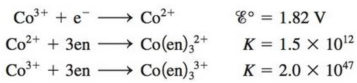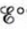Chapter 21, Problem 91CP

Chapter
Section
Textbook Problem

# Consider the following data:where en = ethylenediamine.a. Calculatefor the half-reactionb. Based on your answer to part a, which is the stronger oxidizing agent, Co3+ or Co(en)33+?c. Use the crystal field model to rationalize the result in part b.

(a)

Interpretation Introduction

Interpretation: The standard electrode potential (Eο) for the reaction and stronger oxidizing agent among Co+3 and Co(en)33+ is to be stated. An explanation on basis of the crystal field model corresponding to the fact that one of the given two ions is the stronger oxidizing agent is to be stated.

Concept introduction: The electrons in the d orbital of a transition metal split into high and low energy orbitals when ligands are attached to it. The energy difference between these two levels depends upon the properties of both metal and the ligands. If the ligand is strong, then splitting will be high and the complex will be low spin. If the ligand is weak, then splitting will be less and the complex will be high spin.

To determine: The standard electrode potential (Eο) for the given half reaction.

Explanation

Explanation

The given equations are,

Co+3+eCo+2Eο=1.82V (1)

Co2++3enCo(en)32+K=1.5×1012 (2)

Co3++3enCo(en)33+K2=2.0×1047 (3)

Where,

• Eο is the standard electrode potential for equation (1).
• K is the equilibrium constant of equation (2).
• K2 is the equilibrium constant of equation (3).

The equilibrium constant (K1) for equation (1) is calculated by the formula,

logK1=nEο0.0591

Where,

• K1 is the equilibrium constant of equation (1).
• n is the number of electrons.

The value of n is 1 .

Substitute the value of n and Eο in the above formula.

logK1=1×1.820.0591logK1=30.79K1=6.165×1030

Now the equation (1) becomes,

Co+3+eCo+2K1=6

(b)

Interpretation Introduction

Interpretation: The standard electrode potential (Eο) for the reaction and stronger oxidizing agent among Co+3 and Co(en)33+ is to be stated. An explanation on basis of the crystal field model corresponding to the fact that one of the given two ions is the stronger oxidizing agent is to be stated.

Concept introduction: The electrons in the d orbital of a transition metal split into high and low energy orbitals when ligands are attached to it. The energy difference between these two levels depends upon the properties of both metal and the ligands. If the ligand is strong, then splitting will be high and the complex will be low spin. If the ligand is weak, then splitting will be less and the complex will be high spin.

(c)

Interpretation Introduction

Interpretation: The standard electrode potential (Eο) for the reaction and stronger oxidizing agent among Co+3 and Co(en)33+ is to be stated. An explanation on basis of the crystal field model corresponding to the fact that one of the given two ions is the stronger oxidizing agent is to be stated.

Concept introduction: The electrons in the d orbital of a transition metal split into high and low energy orbitals when ligands are attached to it. The energy difference between these two levels depends upon the properties of both metal and the ligands. If the ligand is strong, then splitting will be high and the complex will be low spin. If the ligand is weak, then splitting will be less and the complex will be high spin.

To determine: The use to crystal field model to rationalise the result in part b.

### Still sussing out bartleby?

Check out a sample textbook solution.

See a sample solution

#### The Solution to Your Study Problems

Bartleby provides explanations to thousands of textbook problems written by our experts, many with advanced degrees!

Get Started